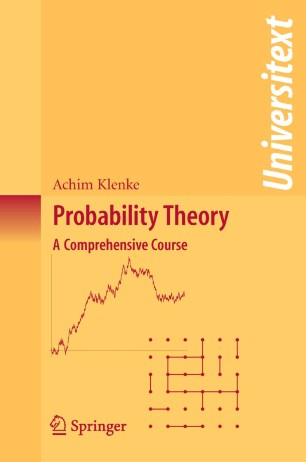# Probability Theory

## A Comprehensive Course

• Achim KlenkeTextbook

Part of the Universitext book series (UTX)

1. Front Matter
Pages I-XII
2. Pages 1-48
3. Pages 49-75
4. Pages 77-84
5. Pages 85-99
6. Pages 101-127
7. Pages 129-142
8. Pages 143-167
9. Pages 169-187
10. Pages 189-203
11. Pages 205-215
12. Pages 231-243
13. Pages 245-270
14. Pages 271-292
15. Pages 327-343
16. Pages 345-378
17. Pages 379-402
18. Pages 403-430
19. Pages 431-445
20. Pages 447-494
21. Pages 495-504
22. Pages 505-524
23. Pages 525-542
24. Pages 543-566
25. Pages 567-590
26. Back Matter
Pages 591-621

### Introduction

Probabilistic concepts play an increasingly important role in mathematics, physics, biology, financial engineering and computer science. They help us to understand magnetism, amorphous media, genetic diversity and the perils of random developments on the financial markets, and they guide us in constructing more efficient algorithms.

This text is a comprehensive course in modern probability theory and its measure-theoretical foundations. Aimed primarily at graduate students and researchers, the book covers a wide variety of topics, many of which are not usually found in introductory textbooks, such as:

• limit theorems for sums of random variables;
• martingales;
• percolation;
• Markov chains and electrical networks;
• construction of stochastic processes;
• Poisson point processes and infinite divisibility;
• large deviation principles and statistical physics;
• Brownian motion; and
• stochastic integral and stochastic differential equations.

The theory is developed rigorously and in a self-contained way, with the chapters on measure theory interlaced with the probabilistic chapters in order to display the power of the abstract concepts in the world of probability theory. In addition, plenty of figures, computer simulations, biographic details of key mathematicians, and a wealth of examples support and enliven the presentation.

### Keywords

Probability theory Random variable common limit theorems measure theory modern probability theory probability statistical physics stochastic processes

#### Authors and affiliations

• Achim Klenke
• 1
1. 1.Institut für MathematikJohannes Gutenberg-Universität MainzMainzGermany

Industry Sectors
Pharma
Biotechnology